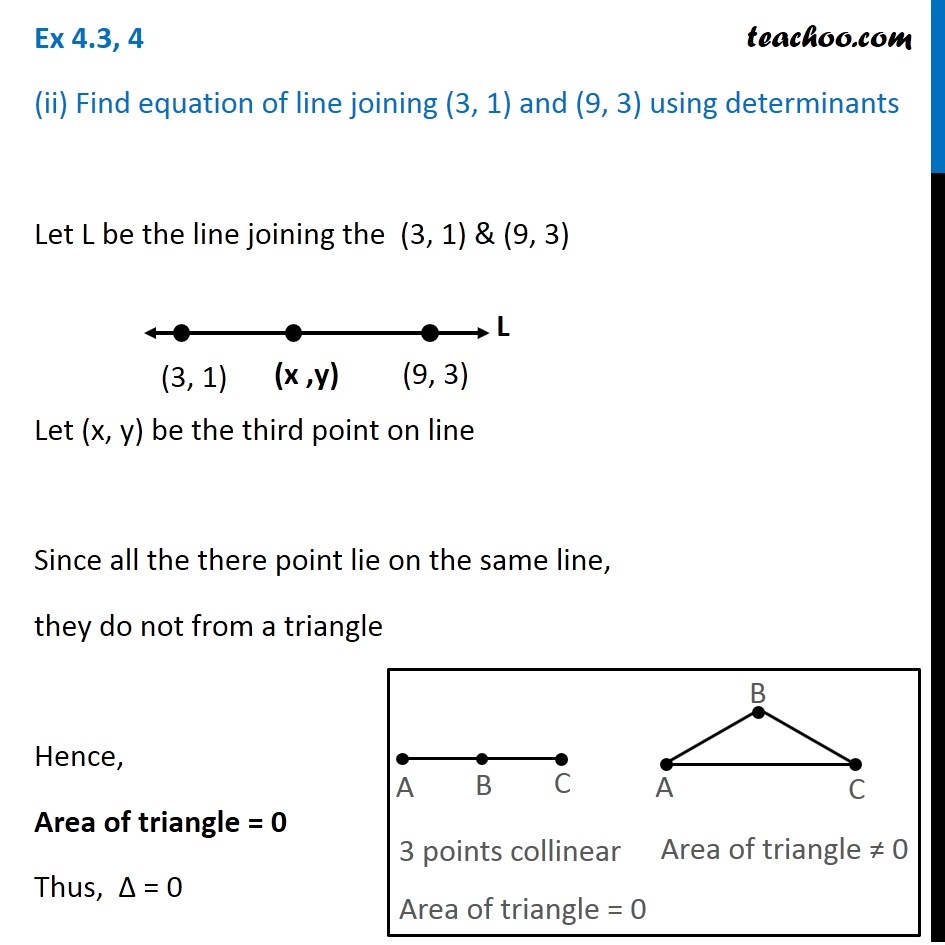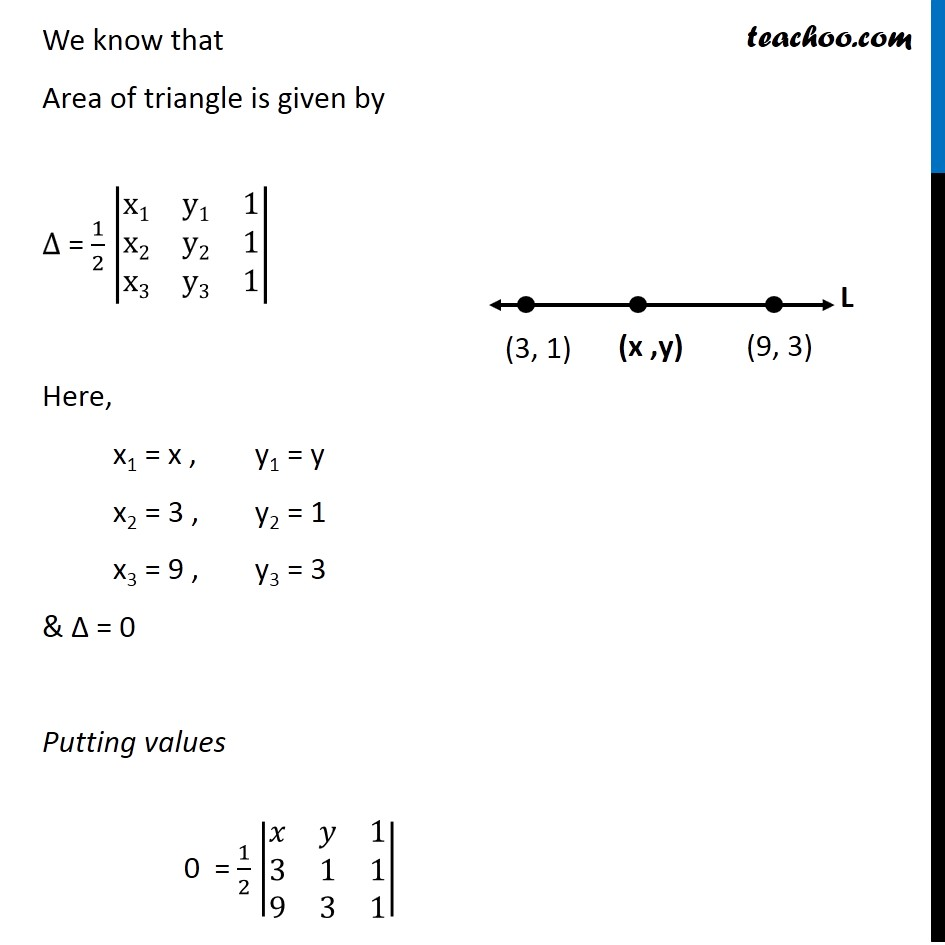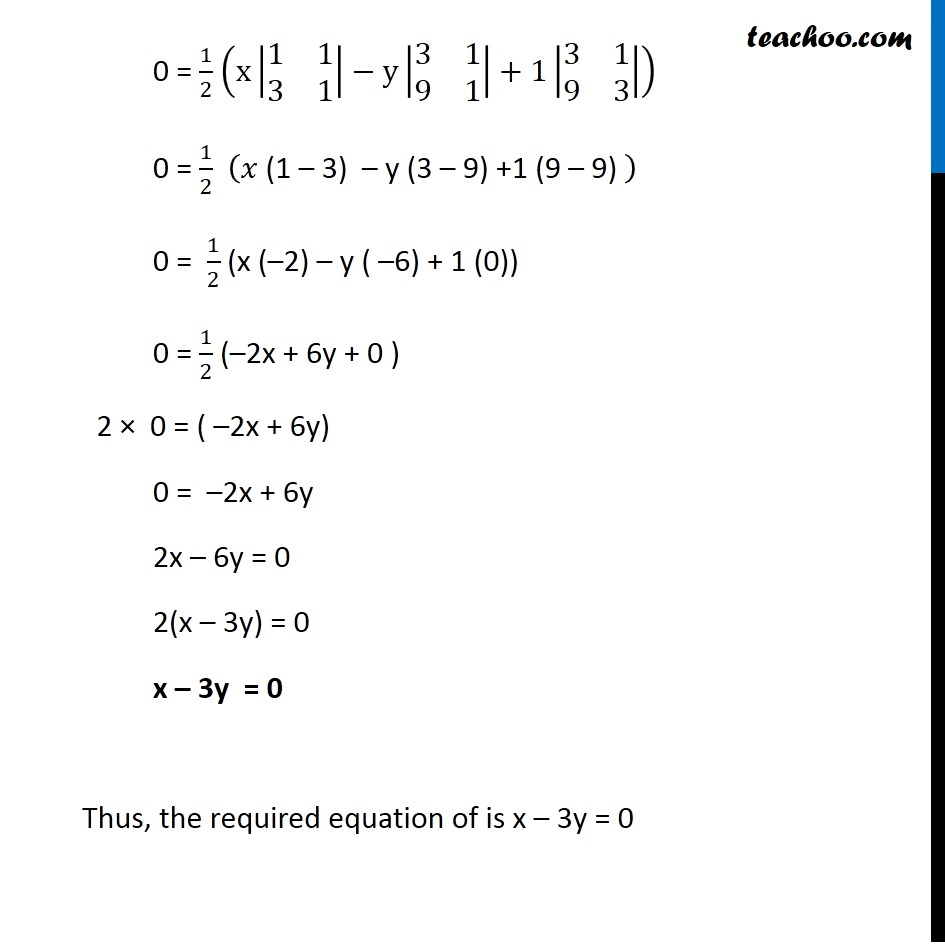Ex 4.3

Chapter 4 Class 12 Determinants
Serial order wiseGet live Maths 1-on-1 Classs - Class 6 to 12

### Transcript

Ex 4.3, 4 (ii) Find equation of line joining (3, 1) and (9, 3) using determinants Let L be the line joining the (3, 1) & (9, 3) Let (x, y) be the third point on line Since all the there point lie on the same line, they do not from a triangle Hence, Area of triangle = 0 Thus, ∆ = 0 We know that Area of triangle is given by ∆ = 1/2 |■8(x1&y1&[email protected]&y2&[email protected]&y3&1)| Here, x1 = x , y1 = y x2 = 3 , y2 = 1 x3 = 9 , y3 = 3 & ∆ = 0 Putting values 0 = 1/2 |■8(𝑥&𝑦&[email protected]&1&[email protected]&3&1)| 0 = 1/2 (x|■8(1&[email protected]&1)|−y|■8(3&[email protected]&1)|+1|■8(3&[email protected]&3)|) 0 = 1/2 (𝑥 "(1 – 3) – y (3 – 9) +1 (9 – 9) " ) 0 = 1/2 (x (–2) – y ( –6) + 1 (0)) 0 = 1/2 (–2x + 6y + 0 ) 2 × 0 = ( –2x + 6y) 0 = –2x + 6y 2x – 6y = 0 2(x – 3y) = 0 x – 3y = 0 Thus, the required equation of is x – 3y = 0